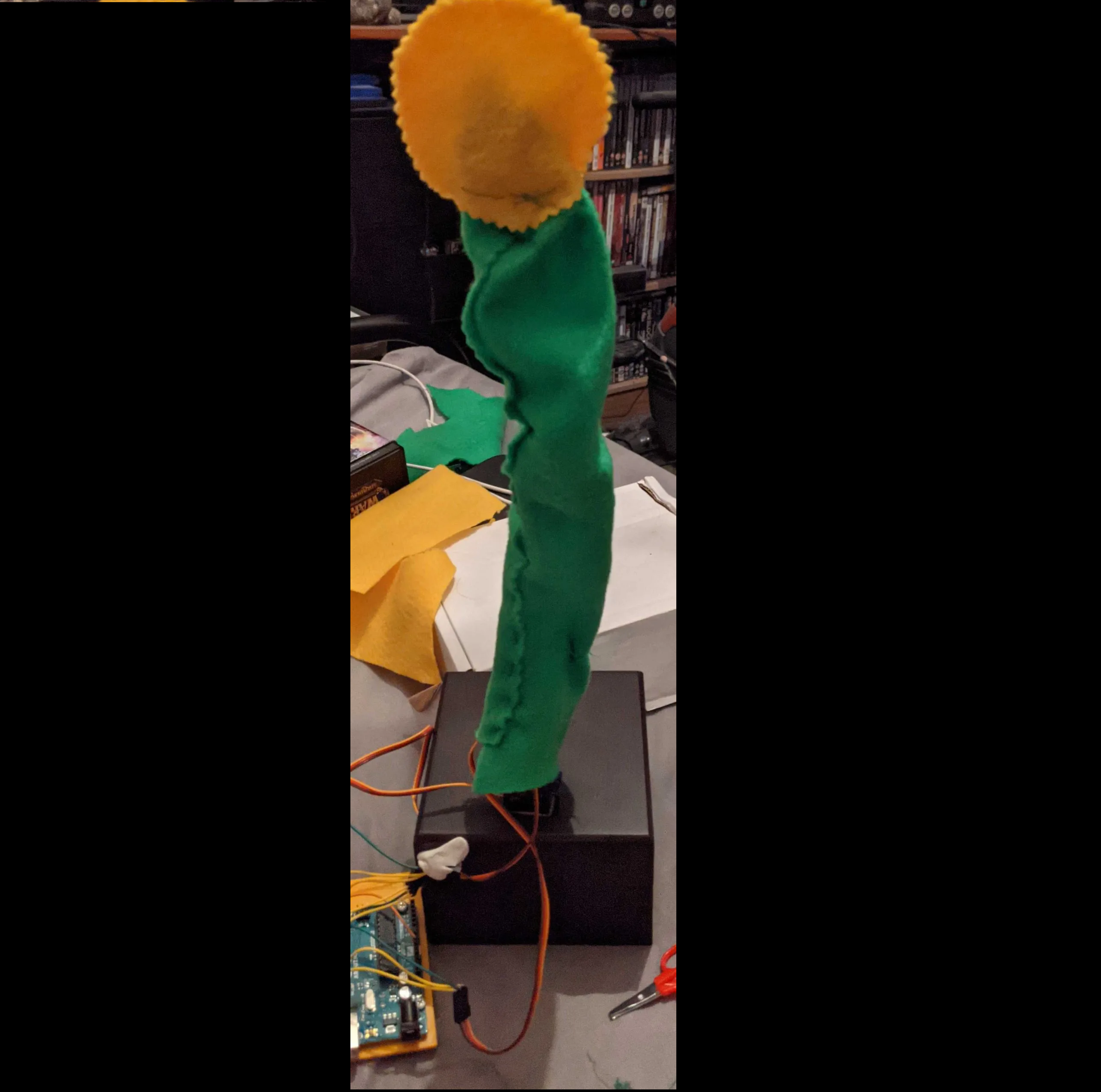# Robo-Flower

So a while ago I got bored and decided to build my first Arduino project since university.

The concept was simple: something that danced back and forth using servos.

The result? A dancing flower.

Using three servos, some pieces of plastic and a few screws that came with them and an Arduino Uno kit along with a watch box some felt and a battery.

I managed to scrap together this:

I think it turned out pretty well!

### Smooth Motion

The problem with servos apparently is that they don’t do smooth motions.

Instead they jerk to whatever position you tell them as quickly as possible.

But a decent workaround is to use a loop to change it’s position slightly again and again with a slight delay.

``````Servo servo3;
void setup()
{
servo3.attach(13);
}
void loop()
{
delay(900);
//  TURN
for (int k = 160; k>= 60; k--) {
servo3.write(k);
delay(3);
}
}``````

### Moving Multiple Servos At Once

Another problem I found was that you couldn’t easily do any kind of multi-threading.

This meant that I couldn’t have one servo go right while another went left.

Luckily to the naked eye moving one servo slightly left and THEN moving the other slightly right over and over looks just about the same.

Thus I ended up with this:

``````// WIGGLE
int k2=120;
for (int i = 60; i <= 160; i++) {
servo1.write(i);
if(k2>80) {
k2--;
}
servo2.write(k2);
delay(3);
}``````

### Full Code

In the end this is the full code that I botched together:

``````#include <Servo.h>

Servo servo1;
Servo servo2;
Servo servo3;
void setup()
{
servo1.attach(11);
servo2.attach(12);
servo3.attach(13);
}
void loop()
{
delay(900);
//  TURN
for (int k = 160; k>= 60; k--) {
servo3.write(k);
delay(3);
}
// WIGGLE
int k2=120;
for (int i = 60; i <= 160; i++) {
servo1.write(i);
if(k2>80) {
k2--;
}
servo2.write(k2);
delay(3);
}

delay(900);

int i2 = 80;
for (int k = 160; k>= 60; k--) {
servo1.write(k);
if(i2<120) {
i2++;
}
servo2.write(i2);
delay(3);
}

delay(900);

// TURN

for (int i = 60; i <= 160; i++) {
servo3.write(i);
delay(3);
}

delay(900);
// WIGGLE

k2=120;
for (int i = 60; i <= 160; i++) {
servo1.write(i);
if(k2>80) {
k2--;
}
servo2.write(k2);
delay(3);
}

delay(900);

i2 = 80;
for (int k = 160; k>= 60; k--) {
servo1.write(k);
if(i2<120) {
i2++;
}
servo2.write(i2);
delay(3);
}

}``````